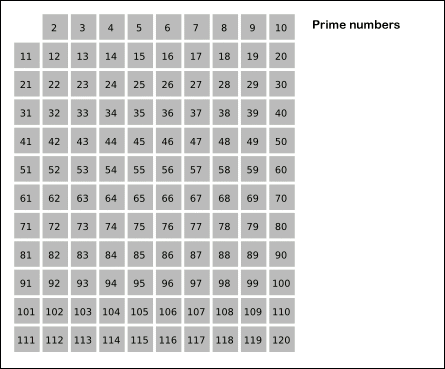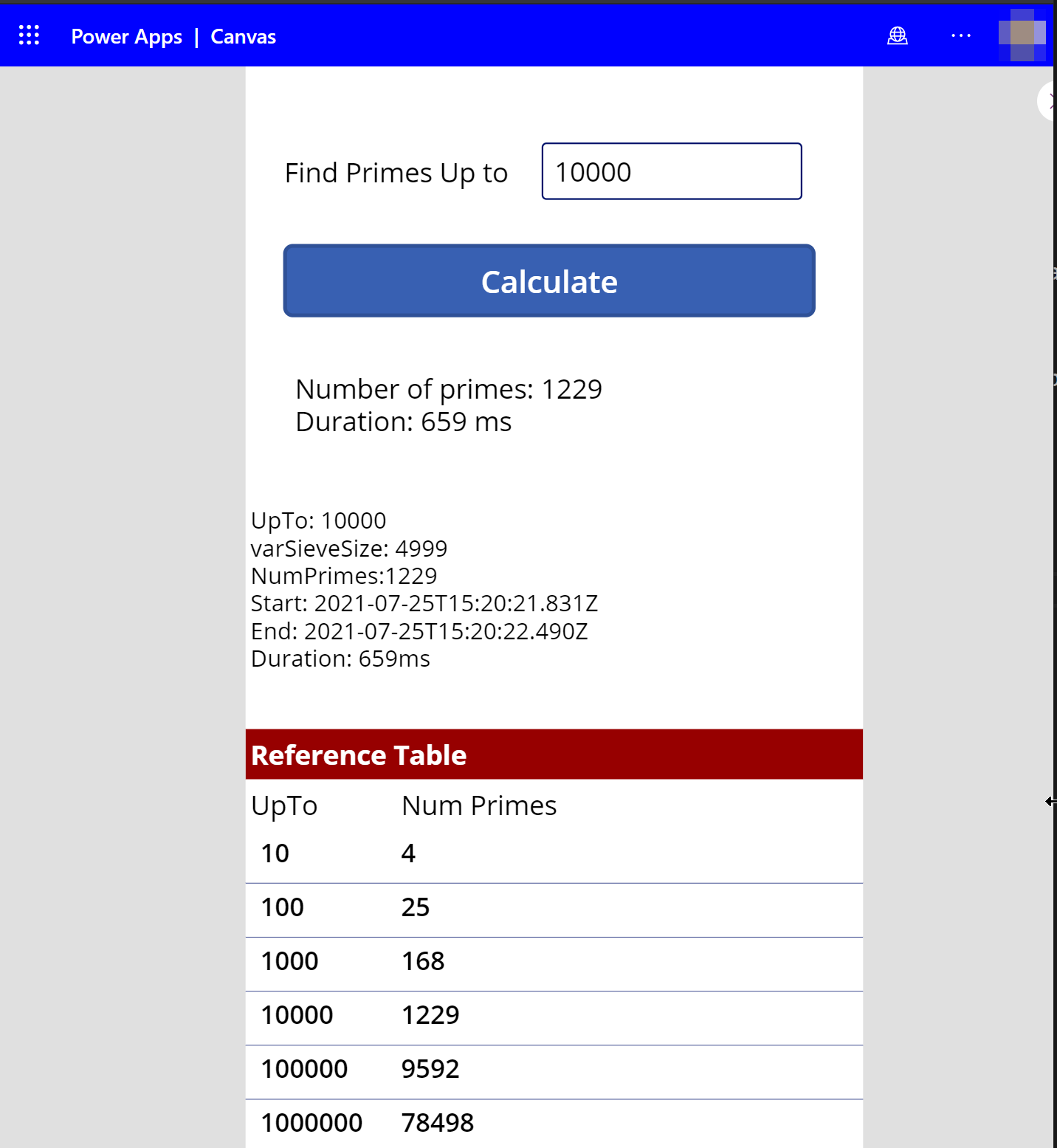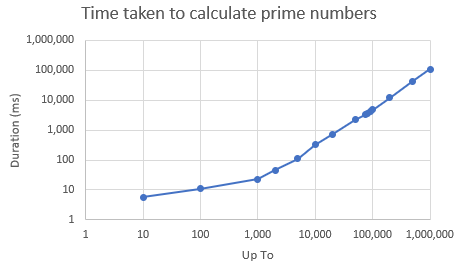# Power Fx Prime Sieve

Dave Plummer is reviewing 50+ different languages to see which can generate prime numbers fastest. Notably absent from the list is Power Fx, lets see if we can change that…

## The challenge

The challenge is to count the number of times all the prime numbers up to one million can be calculated in one second. This is inspired by Dave Plummers initial youtube video drag racing C++, C# and Python.

The drag race has exploded and the Primes github repo now contains over 50 languages, from Assembly though to Zig. There’s even Emojicode 😕!

## The algorithm

Dave uses the Sieve of Erathosthenes method to obtain the primes. It’s explained really well in his video, and also nicely explained by this animation:.

1. We create a collection of all candidate prime numbers upto our target number. 120 in the animation above.
2. We take the first prime number, 2, and then cross off all multiples of the number (4, 6, 8, 10, …)
3. We take the next remaining number, 3, and then cross off all multiples (6, 9, 12, 15, …)
4. We take the next remaining number, 5, and then cross off all multiples (10, 15, 20, 25, …)
5. We take the next remaining number, 7, and then cross off all multiples (14, 21, 28, 35, 42, 49, …)
6. We take the next remaining number, 9, and then cross off all multiples (18, 27, 36, 45, )

We only need to proceed up to the square root of the number being searched, in this case sqrt(120) = 10.9 which rounds down to 10.

1. The remaining numbers in the collection are all prime numbers.

## Capabilities of Power Fx

Can we do this in Power Fx? We’re going to limit ourselves to pure Power Fx. It’d likely be much faster to use a custom connector and Azure function to obtain the prime numbers, but that’s not the point!

Power Fx only has one built-in iterator, the `ForAll()` function. `ForAll` processes records in any order, as the documentation states:

When writing your formula, keep in mind that records can be processed in any order and, when possible, in parallel. The first record of the table may be processed after the last record.

## The Power Fx algorithm

We’re going to follow Dave’s prime sieve algorithm as closely as possible. Firstly we want to create a table containing all the candidate prime numbers upto our chosen limit. We’ll call that table our sieve. As we identify prime numbers we’ll remove multiples of that prime number from the sieve. After we’ve finished processing only prime numbers will remain in the sieve.

So for a limit of 10 our sieve would initially contain: 2, 3, 4, 5, 6, 7, 8, 9, 10.

As an optimisation we’ll not add multiples of 2 to the sieve, so the sieve becomes: 3, 5, 7, 9.

Generating our sieve using Power Fx we can use the `Sequence()` function.

The number of rows in our sieve can be calculated by dividing the limit by 2 and adding 1, e.g.

``````   Set(varSieveSize, RoundDown((cmpCountPrimes.UpTo - 1) / 2, 0));
``````

We can then create a collection of all candiate prime numbers, via:

``````ForAll(
Sequence(varSieveSize),
Collect(colSieve, 1 + (Value * 2))
);
``````

To explain the above:

• `Sequence(varSieveSize)` will return all the numbers from 1 to the sieve size as a table.
• We use `ForAll()` to iterate through each sequence number and call `Collect()`.
• `Collect(colSieve, 1 + Value * 2)` takes the current sequence number (Value), and uses a small formula to calculate the candidate number, then adds it to our collection, colSieve.

We now have a collection, colSieve, with all the candidate prime numbers, e.g. 3, 5, 7, 9

There is one limitation. From the docs:

Sequence is limited to 50,000 records.

This limits the maximum number upto which we can find prime numbers to 100,000. Keep reading, we’ll resolve this later on…

# Filtering the sieve

The next step is to filter the sieve. For each number in the sieve, we’ll look for any multiples and remove them from the sieve.

So for number 3, we find any other numbers that are above 3 and are multiples of three and remove them from the sieve collection.

``````RemoveIf(
colSieve,
ThisRecord.Value > 3,
Mod(ThisRecord.Value, 3) = 0
)
``````

The above removes numbers 6, 9, 12, 15 and so on from the sieve collection.

We can generalise it for number n, and optimise it further by starting at n squared.

``````RemoveIf(
colSieve,
ThisRecord.Value >= n * n,
Mod(ThisRecord.Value, n) = 0
)
``````

Finally, we can generate n using another `Sequence()` and `ForAll()`. We only need to evaluate factors up to the square root of our limit.

``````
Set(varMaxFactor, RoundDown(-1 + RoundUp(Sqrt(cmpCountPrimes.UpTo), 0) / 2, 0));

ForAll(
sequence(varMaxFactors),
With(
{ n: 1 * Value * 2},
RemoveIf(
colSieve,
ThisRecord.Value >= n * n,
Mod(ThisRecord.Value, n) = 0
)
)
)
``````

Explaining the above:

• Power Fx does not allow modification of the collection being iterated upon, so we have to generate another sequence. As per the docs:

Take care to avoid ordering dependencies. For this reason, you can’t use the UpdateContext, Clear, and ClearCollect functions within a ForAll function because they could easily be used to hold variables that would be susceptible to this effect. You can use Collect, but the order in which records are added is undefined.

• The sequence only needs to go up to the square root of our limit upto which we’re searching, which is how we calculate varMaxFactor.

• We recalculate n, the candidate prime for each sequence.

## Optimising further

The additional work to calculate the iterated sequence can be simplified if we use the existing sieve collection and filter it to return just candidate numbers below the square root of our limit.

``````Set(colSourceSieve, Filter(colSieve, Value <= Sqrt(cmpCountPrimes.UpTo)));

// Remove elements from the sieve
ForAll(
colSourceSieve,
With(
{ n: Value },
RemoveIf(
colSieve,
ThisRecord.Value >= Power(n, 2),
Mod(ThisRecord.Value, n) = 0
)
)
);
``````

### Increasing the limit

As mentioned above `Sequence()` will only return a collection up to 50,000 rows. To generate all the primes under 1 million we need to increase the sieve size to 1,000,000 / 2 = 500,000 (!!). To do that we’ll nest sequences within which we’ll continue to add to a giant collection.

``````// ** Nest sequences to generate sieves with more than 50,000 rows **

// Generate prime candidates for whole iterations of 50,000, i.e. 1 .. 50,000, 50,001 .. 100,000
Set(varWholeIterations, RoundDown(varSieveSize / 50000, 0));

// Generate sequence numbers for whole iterations, i.e. 1 .. 50,000, 50,001 .. 100,000
ForAll(
Sequence(varWholeIterations),
With(
{ outer: Value - 1 },
ForAll(
Sequence(50000),
Collect(colSieve, 1 + (((50000 * outer) + Value) * 2));
)
)
);

// Generate remaining prime candidates
ForAll(
Sequence(Mod(varSieveSize, 50000)),
Collect(colSieve, 1 + (((50000 * varWholeIterations) + Value) * 2));
);
``````

Explaining the above:

• We calculate the number of times we’ll need to iterate through 50,000.
• We repeatedly add to the colSieve for each full run of 50,000. The number we add to the sieve is a candidate prime.
• Finally we add the remaining prime candidates for the remainder that is not a multiple of 50,000.

The result of this is a list of prime candidates upto our limit. We’re able to generate prime number candidates up to 2.5 billion (50,000 * 50,000) if time, patience and memory permit. In practice I’ve only tried up to 1,000,000, and you’ll see why below.

### Putting it all together

``````Set(varTimeStart, Now());

// ** Generate the sieve

// Determine the number of rows in the initial sieve
Set(varSieveSize, RoundDown((cmpCountPrimes.UpTo - 1) / 2, 0));

// Clear the sieve from previous any previous runs
Clear(colSieve);

// Determine number of whole iterations of 50,000 required
Set(varWholeIterations, RoundDown(varSieveSize / 50000, 0));

// Generate prime candidates for whole iterations of 50,000, i.e. 1 .. 50,000, 50,001 .. 100,000
ForAll(
Sequence(varWholeIterations),
With(
{ outer: Value - 1 },
ForAll(
Sequence(50000),
Collect(colSieve, 1 + (((50000 * outer) + Value) * 2));
)
)
);

// Generate remaining prime candidates
ForAll(
Sequence(Mod(varSieveSize, 50000)),
Collect(colSieve, 1 + (((50000 * varWholeIterations) + Value) * 2));
);

// ** Filter the sieve

// Copy the sieve so that we can use it as the iterator.  We only need to copy up to the sqrt
Set(colSourceSieve, Filter(colSieve, Value <= Sqrt(cmpCountPrimes.UpTo)));

// Remove elements from the sieve
ForAll(
colSourceSieve,
With(
{ n: Value },
RemoveIf(
colSieve,
ThisRecord.Value >= Power(n, 2),
Mod(ThisRecord.Value, n) = 0
)
)
);

// ** Prime numbers generated

// Add 1 because 2 is a prime number
Set(varNumPrimes, 1 + Count(colSieve));

// Report how long we took
Set(varTimeEnd, Now());
Set(varDuration, DateDiff(varTimeStart, varTimeEnd, Milliseconds));
``````

The above is put into the `OnReset` event in a canvas component. OnReset is triggered by the `OnSelect` event on the Calculate button.### Performance

How fast is it? Firstly, Power Fx isn’t intended to do this! Here’s the results:

Primes UpTo Primes Found Duration (secs)
10 4 0.006
100 25 0.011
1,000 168 0.023
10,000 1,229 3.2
100,000 9,692 4.7
200,000 17,984 12.1
500,000 43,889 43.1
1,000,000 78,498 109Calculating the number of primes up to one million takes 109 seconds, or nearly two minutes!

In Dave’s latest video C# calculates at a rate of 2,046 passes per second.

Our Power Fx algorithm is calculating at 0.0091 passes per second! So C# is approximately 220,000 times faster!

## Source Code and Improvements

The Canvas App and source code is available on my github, here: https://github.com/filcole/PowerFxPrimes

I suspect the implementation could be improved, and if so I’d love suggestions and pull requests.

• Could the sieve be improved by using a large string instead of a collection? Scott Durow used strings instead of collections for the maps in PowerLemmings.
• Is there a way to efficiently flip bits within a hexadecimal string to only use one bit prime candidate? This seems unlikely.

Until Microsoft release a runtime for Power Fx that’s not tied to Canvas Apps we can’t submit this to the language drag race. Perhaps one day it will be possible.

## Conclusions

We were able to make Power Fx calculate the number of prime numbers up to one million! However, we’re pushing Power Fx way past its intended purpose, and there are certainly more optimal methods available by using custom connectors. As always, use the correct tool for the job. What else have we learnt?

• Power Fx is remarkably succinct.
• The number of records in a collection can exceed 50,000, and can be generated using nested `Sequence()` functions.
• `ForAll()` is not delegatable, and its processing order is indeterminate. Its iterator is not modifiable within the `ForAll()` itself.
• Data manipulation with large collections is slow, it’s far better to offload heavy processing using a custom connector.
• We can currently only run Power Fx code within Canvas Apps, but hopefully a runner will be extracted by Microsoft so that Power Fx can be included in the primes drag race project. Probably not a priority for Microsoft!